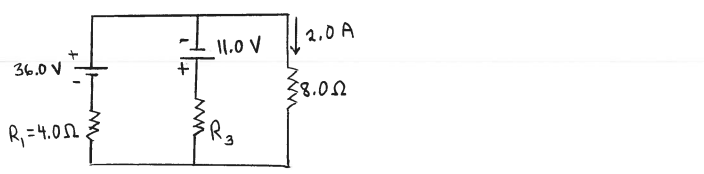# Solution: Consider the circuit shown in the sketch. The current in the 8.0 Ω resistor is 2.0 A, in the direction shown. What is the resistance of the resistor R3?

###### Problem

Consider the circuit shown in the sketch. The current in the 8.0 Ω resistor is 2.0 A, in the direction shown.

What is the resistance of the resistor R3?